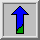# Skeletonization/Medial Axis Transform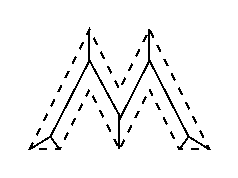Common Names: Skeletonization, Medial axis transform

## Brief Description

Skeletonization is a process for reducing foreground regions in a binary image to a skeletal remnant that largely preserves the extent and connectivity of the original region while throwing away most of the original foreground pixels. To see how this works, imagine that the foreground regions in the input binary image are made of some uniform slow-burning material. Light fires simultaneously at all points along the boundary of this region and watch the fire move into the interior. At points where the fire traveling from two different boundaries meets itself, the fire will extinguish itself and the points at which this happens form the so called `quench line'. This line is the skeleton. Under this definition it is clear that thinning produces a sort of skeleton.

Another way to think about the skeleton is as the loci of centers of bi-tangent circles that fit entirely within the foreground region being considered. Figure 1 illustrates this for a rectangular shape.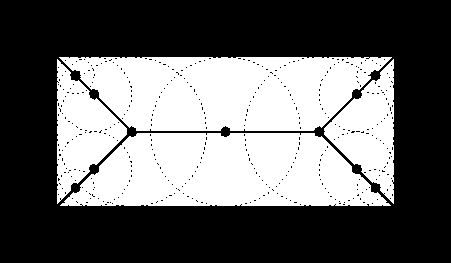Figure 1 Skeleton of a rectangle defined in terms of bi-tangent circles.

The terms medial axis transform (MAT) and skeletonization are often used interchangeably but we will distinguish between them slightly. The skeleton is simply a binary image showing the simple skeleton. The MAT on the other hand is a graylevel image where each point on the skeleton has an intensity which represents its distance to a boundary in the original object.

## How It Works

The skeleton/MAT can be produced in two main ways. The first is to use some kind of morphological thinning that successively erodes away pixels from the boundary (while preserving the end points of line segments) until no more thinning is possible, at which point what is left approximates the skeleton. The alternative method is to first calculate the distance transform of the image. The skeleton then lies along the singularities (i.e. creases or curvature discontinuities) in the distance transform. This latter approach is more suited to calculating the MAT since the MAT is the same as the distance transform but with all points off the skeleton suppressed to zero.

Note: The MAT is often described as being the `locus of local maxima' on the distance transform. This is not really true in any normal sense of the phrase `local maximum'. If the distance transform is displayed as a 3-D surface plot with the third dimension representing the grayvalue, the MAT can be imagined as the ridges on the 3-D surface.

## Guidelines for Use

Just as there are many different types of distance transform there are many types of skeletonization algorithm, all of which produce slightly different results. However, the general effects are all similar, as are the uses to which the skeletons are put.

The skeleton is useful because it provides a simple and compact representation of a shape that preserves many of the topological and size characteristics of the original shape. Thus, for instance, we can get a rough idea of the length of a shape by considering just the end points of the skeleton and finding the maximally separated pair of end points on the skeleton. Similarly, we can distinguish many qualitatively different shapes from one another on the basis of how many `triple points' there are, i.e. points where at least three branches of the skeleton meet.

In addition, to this, the MAT (not the pure skeleton) has the property that it can be used to exactly reconstruct the original shape if necessary.

As with thinning, slight irregularities in a boundary will lead to spurious spurs in the final image which may interfere with recognition processes based on the topological properties of the skeleton. Despurring or pruning can be carried out to remove spurs of less than a certain length but this is not always effective since small perturbations in the boundary of an image can lead to large spurs in the skeleton.

Note that some implementations of skeletonization algorithms produce skeletons that are not guaranteed to be continuous, even if the shape they are derived from is. This is due to the fact that the algorithms must of necessity run on a discrete grid. The MAT is actually the locus of slope discontinuities in the distance transform.

Here are some example skeletons and MATs produced from simple shapes. Note that the MATs have been contrast-stretched in order to make them more visible.

Starting withSkeleton is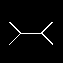MAT isStarting withSkeleton isMAT is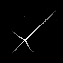Starting withSkeleton is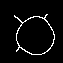MAT is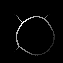Starting with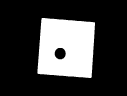Skeleton isMAT isThe skeleton and the MAT are often very sensitive to small changes in the object. If, for example, the above rectangle changes tothe corresponding skeleton becomesUsing a different algorithm which does not guarantee a connected skeleton yieldsSometimes this sensitivity might be useful. Often, however, we need to extract the binary image from a grayscale image. In these cases, it is often difficult to obtain the ideal shape of the object so that the skeleton becomes rather complex. We illustrate this usingTo obtain a binary image we threshold the image at a value of 100, thus obtainingThe skeleton of the binary image, shown in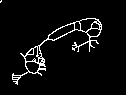is much more complex than the one we would obtain from the ideal shape of the telephone receiver. This example shows that simple thresholding is often not sufficient to produce a useful binary image. Some further processing might be necessary before skeletonizing the image.

The skeleton is also very sensitive to noise. To illustrate this we add some `pepper noise' to the above rectangle, thus obtainingAs can be seen inthe corresponding skeleton connects each noise point to the skeleton obtained from the noise free image.

## Common Variants

It is also possible to skeletonize the background as opposed to the foreground of an image. This idea is closely related to the dual of the distance transform mentioned in the thickening worksheet. This skeleton is often called the SKIZ (Skeleton by Influence Zones).

## Interactive Experimentation

You can interactively experiment with the Skeleton operator by clicking here.

You can interactively experiment with the Medial Axis Transformation operator by clicking here.

## Exercises

1. What would the skeleton of a perfect circular disk look like?

2. Why does the skeleton oflook so strange? Can you say anything general about the effect of holes in a shape on the skeleton of that shape?

3. Try to improve the binary image of the telephone receiver so that its skeleton becomes less complex and better represents the shape of the receiver.

4. How can the MAT be used to reconstruct the original shape of the region it was derived from?

5. Does a skeleton always go right to the edge of the shape it represents?

## References

D. Ballard and C. Brown Computer Vision, Prentice-Hall, 1982, Chap. 8.

E. Davies Machine Vision: Theory, Algorithms and Practicalities, Academic Press, 1990, pp 149 - 161.

R. Haralick and L. Shapiro Computer and Robot Vision, Vol. 1, Addison-Wesley Publishing Company, 1992, Chap. 5.

A. Jain Fundamentals of Digital Image Processing, Prentice-Hall, 1989, Chap. 9.

## Local Information